• 用C写的仿射变换加密解密程序，程序过程很简单，不足之处，欢迎提出c++
• 仿射变换加密

2011-12-14 14:48:50
密码学里面的仿射密码java文件代码 System.out.print("请输入你要加密的偏移量(k):"); t=input(); k=Integer.parseInt(t); System.out.print("请输入明码的倍乘因子(a)："); t=input(); a=Integer....
• 2、解密 解密函数： D© = k1^-1(c - k2)(mod 26) —>k1^-1是k1的乘法逆元素 重点：求k1的乘法逆元素 3、破译 要求：已知密文，求明文 未知：密钥 k1、k2 k1与26互为质数，且k1<26 故，k1可穷举，1,3,5,7,9,11...

1、加密

加密函数：

E(m) = (k1 * m + k2)mod 26 ---->(以英文为载体，有26个字母）
注意：其中k1与26互为质数

2、解密

解密函数：

D© = k1^-1(c - k2)(mod 26)
—> k1^-1是k1的乘法逆元素
重点：求k1的乘法逆元素

乘法逆元素：要求在0,1,2,3,4，…，25找一个数，这个数和k1相乘再取模26运算，结果为1。

3、破译

要求：已知密文，求明文
未知：密钥 k1、k2
k1与26互为质数，且k1<26
故，k1可穷举，1,3,5,7,9,11,15,17,19,21,23,25
k2为正整数，且k2<26.
所以暴力破译后，有12*26 = 312 种情况

Java代码实现：

package com.qul.java1;

import java.util.Scanner;

/**
* @author Dxkstart
* @create 2021-05-25 15:21
*/
public class AffineTest {
public static void main(String[] args) {

Scanner scanner = new Scanner(System.in);

System.out.println("1.*****加密*****");
System.out.println("2.*****解密*****");
System.out.println("3.*****破译*****");
System.out.println("4.*****退出*****");
boolean b = true;
while (b) {
System.out.println("请选择功能：");
int num = scanner.nextInt();

switch (num) {
case 1:
new Encryption().encryption();
break;
case 2:
new Decryption().decryption();
break;
case 3:
new Decode().decode();
break;
case 4:
b = false;
}
}

}

}

//加密算法
class Encryption {
String cleartext;//明文
int k1;//k1与26互为质数
int k2;

public void encryption() {
Scanner scanner = new Scanner(System.in);
scanner.useDelimiter("\n");

System.out.println("请输入k1、k2：");
k1 = scanner.nextInt();
k2 = scanner.nextInt();

System.out.println("请输入明文(小写字符)：");
cleartext = scanner.next();
System.out.println("密文为：");

char[] chars = cleartext.toCharArray();//String转char数组
for (int i = 0; i < chars.length; i++) {
int q = (int) chars[i];//转ACSll码 97-122
if (q != 32) {
int m = (k1 * (q - 97) + k2 % 26) % 26;
char c = (char) (m + 65);//转大写字符
System.out.print(c);
} else {
System.out.print(" ");
}
}
System.out.println();
}
}

//解密算法
class Decryption {
String ciphertext;//密文
int k1;//k1与26互为质数
int k2;

public void decryption() {
Scanner scanner = new Scanner(System.in);
scanner.useDelimiter("\n");//可识别空格字符

System.out.println("请输入k1、k2：");
k1 = scanner.nextInt();
k2 = scanner.nextInt();

System.out.println("请输入密文(大写字符)：");
ciphertext = scanner.next();
System.out.println("明文为：");

//求k1的乘法逆元素
int M;
for (M = 0; M < 26; M++) {
if ((k1 * M) % 26 == 1) {
break;
}
}

char[] chars = ciphertext.toCharArray();
for (int i = 0; i < chars.length; i++) {
int q = (int) chars[i];//转ACSll码 65-90
if (q != 32) {
int m = (M * ((q - 65 + 26) - k2)) % 26;
char c = (char) (m + 97);//转小写字符
System.out.print(c);
} else {
System.out.print(" ");
}
}
System.out.println();
}
}

//破译算法
class Decode {
String ciphertext;//密文
int k1;//k1与26互为质数
int k2;

public void decode() {
Scanner scanner = new Scanner(System.in);
scanner.useDelimiter("\n");

System.out.println("请输入密文(大写字符)：");
ciphertext = scanner.next();
System.out.println("明文为：");

//求k1、k2
for (k1 = 1; k1 <= 26; k1 += 2) {
if (k1 % 13 != 0) {
for (k2 = 1; k2 < 26; k2++) {
//求k1的乘法逆元素
int M;
for (M = 0; M < 26; M++) {
if ((k1 * M) % 26 == 1) {
break;
}
}

char[] chars = ciphertext.toCharArray();
for (int i = 0; i < chars.length; i++) {
int q = (int) chars[i];//转ACSll码 65-90
if (q != 32) {
int m = (M * ((q - 65 + 26) - k2)) % 26;
char c = (char) (m + 97);//转小写字符
System.out.print(c);
} else {
System.out.print(" ");
}
}
System.out.print("     *****    k1 = " + k1 + "  k2= " + k2);
System.out.println();
}
}

}

}

}

举例：

加密：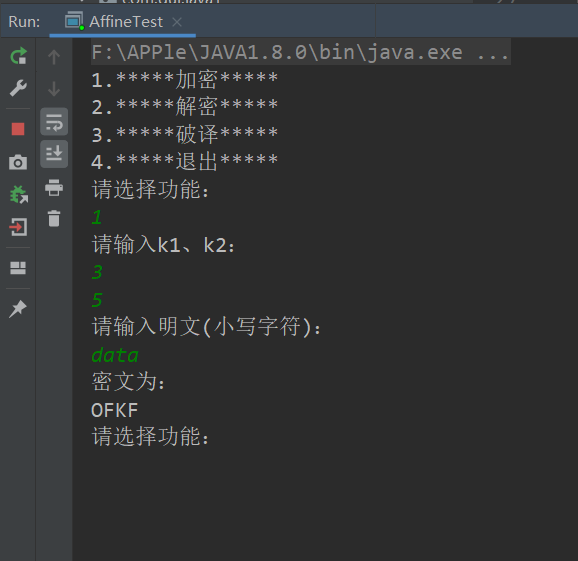解密：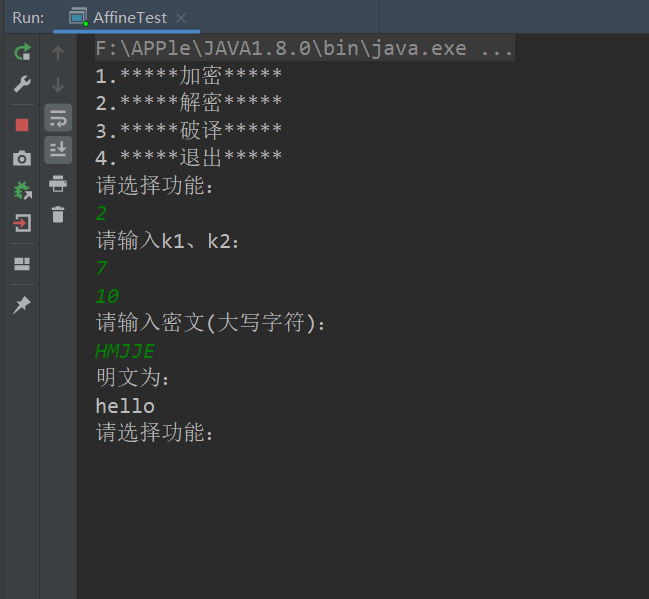破译：

找出有真正意义的句子
破译结果：algorithms are quite general definitions of arithmetic processes
K1 = 3 k2 = 5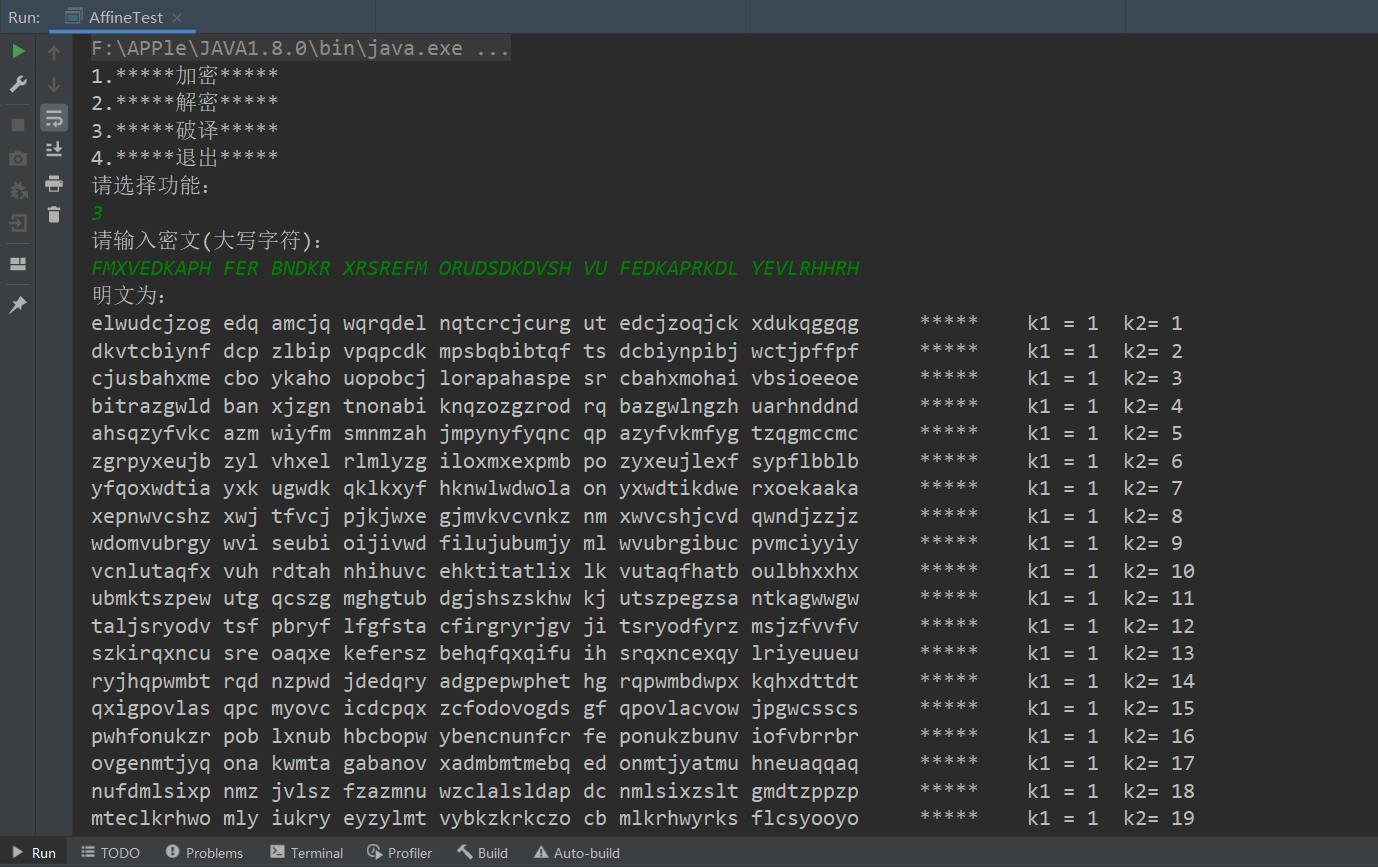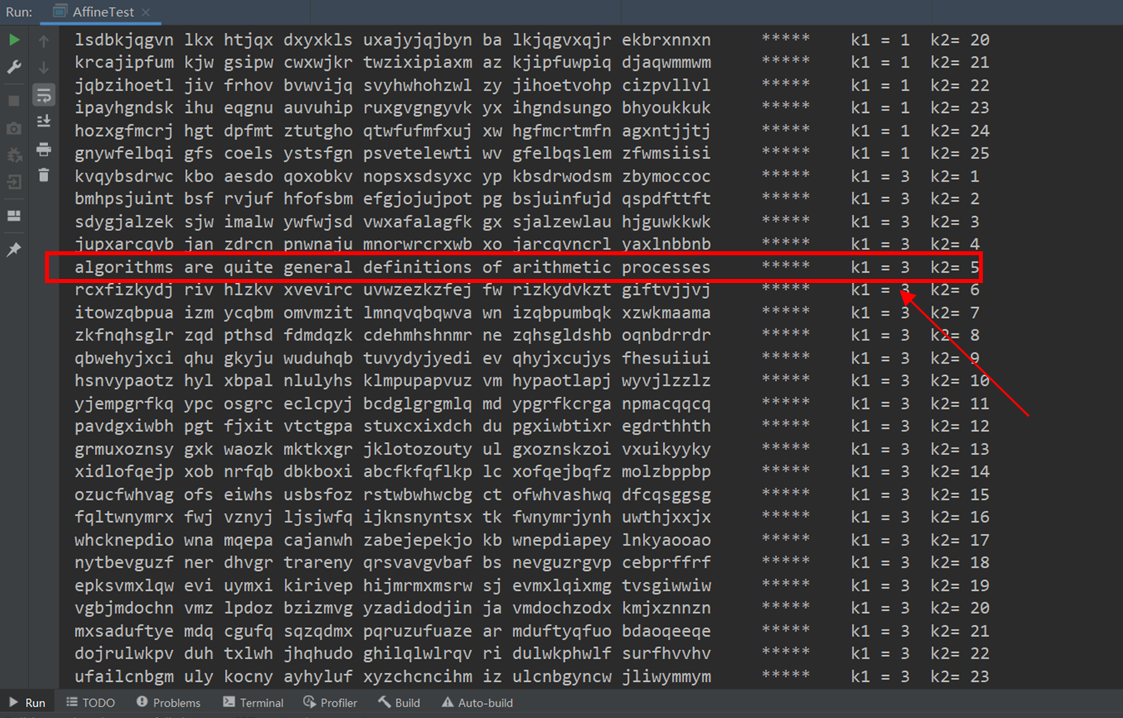展开全文加密解密 java
• 移位密码、仿射变换加解密

前言

这个代码是很久之前的，已经忘记具体的思路了，你可以结合此文章来了解两个加密方式的内容。
前两个步骤是对应加密方式的函数，第三个步骤是整合。

一、移位密码

def yiwei(string,number):
'''
将字符串的每个字母，向右移动指定的位数，并返回移动后的字符串
如：A 向右移动3位，返回值为D
string为字符串，number为整型
A的ASCII码为65
'''
new_string = ""
string = string.upper()  #转换为大写
for i in string:        #移位操作
#转换为ascii码后，经过移位变为新的ascii，再转换回字母
new_string += chr((ord(i)-65+number)%26+65)
return new_string
##print(yiwei('AAA',3)) #测试函数正确性

def yiwei_decode(c):
'''移位密码解密，并打印解密的结果'''
for i in range(1,27): #穷尽密钥搜索，遍历范围[1,26]
print(yiwei(c,i))
print("解密结束")
##yiwei_decode("A") #测试函数正确性

二、仿射变换

def fangshe_key(m,c):
'''
通过已知的两对明文密文，求解得到两个密钥
m为明文,c为密文
[A-Z]转换为数字为[0-25]
'''
#大写
m = m.upper()
c = c.upper()
#切片
m1 = m[:1:]     #明文的第一个字母
m2 = m[-1::]    #明文的最后一个字母
c1 = c[:1:]     #密文的第一个字母
c2 = c[-1::]    #密文的最后一个字母
##    print(m1,m2)
##    print(c1,c2)
#转换为整数
m1 = ord(m1)-65
m2 = ord(m2)-65
c1 = ord(c1)-65
c2 = ord(c2)-65
##    print(m1,m2)
##    print(c1,c2)
#穷举法
for i in range(0,26):
for j in range(0,26):
if (m1*i+j)%26==c1 and (m2*i+j)%26==c2: #同时满足加密算法时
return i,j
return False
##print(fangshe_key("IF","ED")) #测试函数正确性

def fangshe_reverse(x,y):
'''
计算乘法逆元,求解x^-1(%y)的乘法逆元,并返回对应值
'''
i = 0
while True:
if x*i%26==1: #符合乘法逆元条件时
return i
i += 1
##print(fangshe_reverse(9,26)) #测试函数正确性

def fangshe_decode(c,a,b):
'''
仿射变换解密，并打印解密的结果
c为密文，a和b为密钥
0<=a,b<=25，且满足gcd(a,26)=1，a^-1表示a的逆元
加密公式：c = a*m + b%26
解密公式：m = (a^-1)*(c-b)%26
'''
new_string = ''
c = c.upper() #大写转换
for i in c: #逐个字母解密
new_i = ord(i)-65 #转换成数字
new_i = (fangshe_reverse(a,26)*(new_i - b))%26 #解密
new_string += chr(new_i + 65) #转换回大写字母
print(new_string)
print("解密结束")
##fangshe_decode("ED",9,10) #测试函数正确性

三、全部代码

移位密码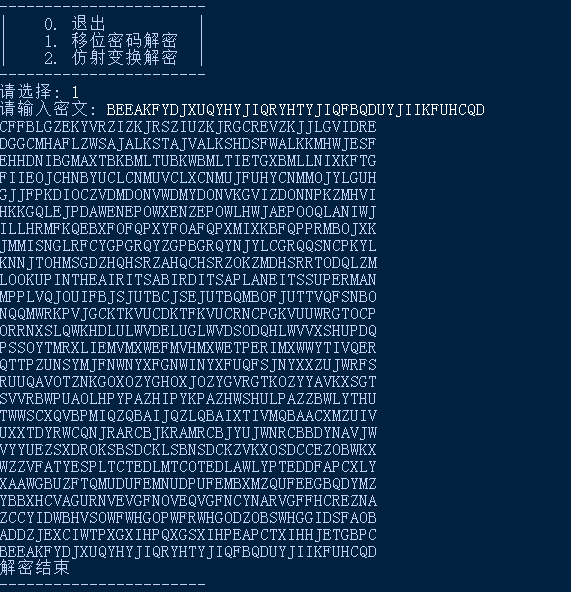仿射变换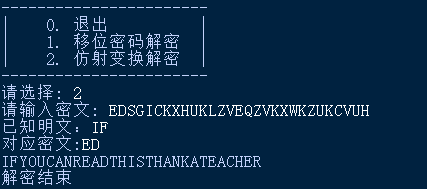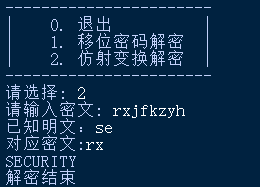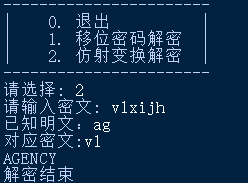# coding=utf-8
# 作者：小狐狸FM
# 题目：古典密码
'''
菜单界面
'''
print("-----------------------")
print("|    0. 退出          |")
print("|    1. 移位密码解密  |")
print("|    2. 仿射变换解密  |")
print("-----------------------")

def yiwei(string,number):
'''
将字符串的每个字母，向右移动指定的位数，并返回移动后的字符串
如：A 向右移动3位，返回值为D
string为字符串，number为整型
A的ASCII码为65
'''
new_string = ""
string = string.upper()  #转换为大写
for i in string:        #移位操作
#转换为ascii码后，经过移位变为新的ascii，再转换回字母
new_string += chr((ord(i)-65+number)%26+65)
return new_string
##print(yiwei('AAA',3)) #测试函数正确性

def yiwei_decode(c):
'''移位密码解密，并打印解密的结果'''
for i in range(1,27): #穷尽密钥搜索，遍历范围[1,26]
print(yiwei(c,i))
print("解密结束")
##yiwei_decode("A") #测试函数正确性

def fangshe_key(m,c):
'''
通过已知的两对明文密文，求解得到两个密钥
m为明文,c为密文
[A-Z]转换为数字为[0-25]
'''
#大写
m = m.upper()
c = c.upper()
#切片
m1 = m[:1:]     #明文的第一个字母
m2 = m[-1::]    #明文的最后一个字母
c1 = c[:1:]     #密文的第一个字母
c2 = c[-1::]    #密文的最后一个字母
##    print(m1,m2)
##    print(c1,c2)
#转换为整数
m1 = ord(m1)-65
m2 = ord(m2)-65
c1 = ord(c1)-65
c2 = ord(c2)-65
##    print(m1,m2)
##    print(c1,c2)
#穷举法
for i in range(0,26):
for j in range(0,26):
if (m1*i+j)%26==c1 and (m2*i+j)%26==c2: #同时满足加密算法时
return i,j
return False
##print(fangshe_key("IF","ED")) #测试函数正确性

def fangshe_reverse(x,y):
'''
计算乘法逆元,求解x^-1(%y)的乘法逆元,并返回对应值
'''
i = 0
while True:
if x*i%26==1: #符合乘法逆元条件时
return i
i += 1
##print(fangshe_reverse(9,26)) #测试函数正确性

def fangshe_decode(c,a,b):
'''
仿射变换解密，并打印解密的结果
c为密文，a和b为密钥
0<=a,b<=25，且满足gcd(a,26)=1，a^-1表示a的逆元
加密公式：c = a*m + b%26
解密公式：m = (a^-1)*(c-b)%26
'''
new_string = ''
c = c.upper() #大写转换
for i in c: #逐个字母解密
new_i = ord(i)-65 #转换成数字
new_i = (fangshe_reverse(a,26)*(new_i - b))%26 #解密
new_string += chr(new_i + 65) #转换回大写字母
print(new_string)
print("解密结束")
##fangshe_decode("ED",9,10) #测试函数正确性

if __name__=='__main__':
while True:
choose = int(input("请选择: "))
if choose==1:
string = input("请输入密文: ")
yiwei_decode(string)
elif choose==2:
string = input("请输入密文: ")
m = input("已知明文：")
c = input("对应密文:")
a,b = fangshe_key(m,c)
fangshe_decode(string,a,b)
else:
break

展开全文python 加密解密 移位密码 仿射
• 基于C语言，实现仿射密码算法，随机生成密钥a、b，加密和解密程序对任意满足条件的a、b都能够进行处理。
• 前言：摘抄自wiki的关于仿射变换的定义：仿射变换，又称仿射映射，是指在几何中，一个向量空间进行一次线性变换并接上一个平移，变换为另一个向量空间。一个对向量平移，与旋转放大缩小的仿射映射为 【1】1、移位...

前言：

摘抄自wiki的关于仿射变换的定义：

仿射变换，又称仿射映射，是指在几何中，一个向量空间进行一次线性变换并接上一个平移，变换为另一个向量空间。

一个对向量平移，与旋转放大缩小的仿射映射为【1】

1、移位加密：

比方说：把字母表全部向右循环移1位，也就是A变成B，B变成C，... ，

Z变成A。

用数学一点的术语我觉得所谓“移位”就是映射吧。

那么我们可以写出通解公式，——现在是已知明文和加密步骤，进行加密，也就是求密文。

New = (Old + k) (mod table)

【2】

注释：

New：要求的密文

Old  ：明文

k ：移位的位数

table：这张表有多大，比方说字母表就是26个字母，table就是26.

2、仿射变换：

移位加密和仿射变换有啥关系呢？

额 ，因为移位加密就是一种放射变换。。。

首先我们在1里面的变量命名现在改一下，以便更好地认识，变成：

y

= (x + b)(mod m) 【3】

是不是和【1】很像啦，可惜我们的x没有系数。。

但是没关系，我们完全可以自己加上一个系数，把【3】变成：

y

= (ax + b)(mod m)   a,b为整数； 【4】

这样已经很像了，只不过【1】里面的自变量是向量。

但是，我们这里讨论的是一维的变换，所以不用用到2维及其以上的向量。(一维数字标记的向量就是普通的数嘛……)

3、知道了1、2这些，我们现在的目标就是——

"当知道一个一维的仿射变换的加密，

a. 怎么把明文加密

b.

怎么把密文解密 "

对于"a.

怎么把明文加密"，我们已经解决了，就是【4】那个公式。

对于"b.

怎么把密文解密"嘛，其实也不复杂。待我不快不慢地说来。

首先由【4】我们可以得到什么？

没错！就是 y

≡ (ax + b)(mod m) —— 这是明显的事实不用证明了。

然后正视一下题目是什么：我们现在已知a,b,y,m，要求x。

1). 左右移位一下 ,变为y-b

≡ (ax)(mod m) 【5】

{为啥【5】是对的？

首先同余式可以相加，

即 若 a ≡ b (mod m),c ≡ d (mod m)， 【*】

那么(a +

c) ≡ (b + d)(mod m) .

——同余式可以相加的证明用同余的定义就好……

然后  y ≡ (ax +

b)(mod m)

-b ≡ -b (mod

m)

所以……

}

2). 已知y-b

≡ (ax)(mod m)，x未知，求x.

现在已知ax ≡(y-b) (mod

m)；

{首先我们要知道 同余式可以相乘，若【*】，则 ac

≡ bd (mod m) .证明同样可以基于同余定义。}

这时候我们就脑补了，如果有一个c能使得

cax ≡ x ≡ c(y-b) (mod m)就好了！

也就是说这个c如果能使得ca = 1或者 更宽一点的：ca ≡ 1(mod

m).那么就解决了！

3). 于是问题变成了：找一个c使得，ca ≡ 1(mod

m)

于是很容易联系到费马小定理、欧拉定理一类的。

但是费马小定理要求m一定要是素数，这样和我们题目不符。

所以看看欧拉定理，对 gcd(a,m) =

1(m>1)，有aΦ(m)≡ 1 (mod m)

{Φ(m) 为欧拉函数，就是小于等于m的与m互素的正整数个数。如Φ(2) = 1

，Φ(6) = 2——6与{1,5}互素。}

所以如果ca

= aΦ(m)，那么就有ca ≡ 1(mod m)。

于是c

= aΦ(m)-1. 但是gcd(a,m) = 1，这一点不能漏。

4、综上，我们发现了，当c

= aΦ(m)-1时 ；

cax ≡c(y-b) (mod m)

化为 x ≡c(y-b) (mod m) {现在要附加gcd(a,m)=1这个条件了！}

5、那么对于仿射变换，

我们知道变化规则[即位移b]后，如何把密文[y]翻译成明文[x]呢？

结论是：

step

1.  求 c

= aΦ(m)-1

step 2.  x ≡c(y-b) (mod m)

番外小剧场：

窝：你在2里面为啥要改名字？该问题纯属好奇！

big窝：此中原因有2——

1. 如文中所说，为了更直观地比较。

2.

也是最根本的原因——变量命名如此之平凡，根本不是我的feel！OK？

窝：......

窝：第二个问题——你怎么知道同余式符合相加相乘原理？你怎么想到的？

big窝：

首先"同余式符合相加相乘原理"这个结论在数论里就像是实数有相加相乘的运算一样自然，如果想不到那么你需要找一本数论的书看看，不需要看完这就足以变成你的常识。至于怎么想到的，如果给你一堆数，你能想到的最基本的运算就是做加减乘除了吧？

窝：恩……好像是的……

big窝：那不就得了，说了同余式符合相加相乘原理是同余式的基本运算。

窝：……哦……

原文：http://www.cnblogs.com/PeanutPrince/p/3543723.html

展开全文• 仿射加密加解密算法

千次阅读 2018-03-31 16:16:17
概述：基本上和数学上的仿射变换类似y=ax+b，通过如此达到一一对应加密。仿射变换加密加密过程：加密算法：c=a*m+ b(mod n)加密过程:1.获取a，b（密钥），n（字符个数）2.获取明文。3.加密成密文，明文转换成各个...

概述：基本上和数学上的仿射变换类似
y=ax+b，通过如此达到一一对应加密。

仿射变换加密加密过程：
加密算法：c=a*m+ b(mod n)

加密过程:
1.获取a，b（密钥），n（字符个数）

2.获取明文。
3.加密成密文，明文转换成各个字符所对应的数字，将所得数字带入上面的算法公式，得到数字再转换成对应的字符

解密过程：
算法：m=a^-1（m-b）（mod n）这里a^-1不是指倒数，而是a关于字符数量模的乘法可逆元，下面介绍一下乘法可逆元

乘法可逆元定义；
群G中任意一个元素a,都在G中有唯一的逆元a‘,具有性质aa'=a'a=e,其中e为群的单位元
这个官方的定义不学数论什么肯定看不太懂。。没事我们只研究数学应用，不学理论，

举个例子好了：
4关于模7的乘法逆元为多少?

可令4X≡1mod7，即可等价于4X=7K+1，其中X,K为整数，求X和K。
由此看出可逆元的通俗定义

如果ax≡1modf，那么a为关于模f的乘法逆元。
另外也有条件，当a与f互素时，a关于模f的乘法逆元有解。如果不互素，则无解。如果f为素数，则从1到f-1的任意数都与f互素，即在1到f-1之间都恰好有一个关于模f的乘法逆元。

再来一个更具体的例子，
求5关于14的乘法逆元

用辗转相除法
14=5*2+4

5=4*1+1
反过来写
1=5-4=5-（14-5*2）=5*3-14

因此5关于模14的乘法逆元为3.
如此即可求出逆元，然后再带入公式即可实现解密。

下面给出仿射加密算法的算法实现。（直观起见采用C++）

#include<iostream>
using namespace std;
#define N 26  //这里只采用26个字母的加解密

//加密
char *encry(char *Plain, int a, int b, int n);
char *decry(char *Cipher, int a, int b, int n);
//获取可行仿射加密的a值数组
void setArr(int Canuse[], int n);
//求GCD（最大公约数）
int Gcd(int a, int b);
//求a关于模n的乘法可逆元
int Multiplicative_inverse_modulo(int Canuse[], int a, int n);
int main() {
int a, b;
char str = "";
cout << ("输入密钥a，b的值") << endl;
cin >> a >> b;
cout << "输入明文内容" << endl;
cin >> str;
cout << "明文为" << endl;
cout << str << endl;
//加密
encry(str, a, b, N);
//输出密文
cout << str << endl;
//解密
decry(str, a, b, N);
//输出解密内容
cout << str << endl;
return 0;
}
//加密函数实现
char *encry(char *Plain, int a, int b, int n)
{
char *tmp = Plain;
if (Plain == NULL)return NULL;
while (*Plain) {
if (' ' == *Plain)
{
++Plain;
continue;
}
if ((*Plain < 'A') || (*Plain > 'Z'))
return NULL;
*Plain -= 'A';
*Plain = (a*(*Plain) + b) % n;
*Plain += 'A';
++Plain;
}
return tmp;
}
//解密所需基础算法实现
void setArr(int Canuse[], int n) {
for (int i = 1; i < n; i++) {
if (1 == Gcd(n, i))
*(Canuse++) = i;
}
}
int Gcd(int a, int b) {
int gcd = 0;
int div = 0;
//辗转相除法
do {
div = a%b;
gcd = b;
a = b;
b = div;
} while (div);
return gcd;
}
//求乘法可逆元
int Multiplicative_inverse_modulo(int Canuse[], int a, int n) {
for (int i = 0; Canuse[i] != 0; i++) {
if (1 == (a*Canuse[i]) % n)
return Canuse[i];
}
return 0;
}
//解密实现
char *decry(char *Cipher, int a, int b, int n) {
char *tmp = Cipher;
int Canuse = { 0 };//符合条件的a值
int moda = 0;//a的乘法可逆元
int i = 0;
if (Cipher == NULL)return NULL;
for (; i < 32; i++)Canuse[i] = 0;
setArr(Canuse, n);//存放符合条件的a.
moda = Multiplicative_inverse_modulo(Canuse, a, n);
while (*Cipher) {
if (' ' == *Cipher) {
++Cipher;
continue;
}
if ((*Cipher < 'A') || (*Cipher > 'Z'))
return NULL;
*Cipher -= 'A';
*Cipher = (moda*(*Cipher - b + n)) % n;
*Cipher += 'A';
++Cipher;
}
return tmp;
}
只恨数学学的不够多啊。
展开全文MIMA
• 一个仿射变换加密的Matlab程序源代码，通过设定的密钥参数k1、k2对给定的明文进行加密得到相应的密文。附带TXT文本代码。
• 对于仿射密码算法过程这里不做过多讲解，请自行参照教材或者网络资料。这里需要注意的是，默认为...下面是用python实现的加解密过程： 密钥为k=（11,4） （1）加密过程 （2）解密过程，密钥k的逆元求出后直接带到公式python 加密 算法 仿射密码
• 2、用仿射变换7x+10解密如下密文：HMJJE 3、用仿射变换破译如下密文： FMXVEDKAPHFERBNDKRXRSREFMORUDSDKDVSHVUFEDKAPRKDLYEVLRHHRH #include #include<string.h> using namespace std; int main(){ int k1, ...加密解密
• 1）用C\C++语言实现单表仿射（Affine）/解密算法； 2）用C\C++语言实现统计26个英文字母出现频率的程序； 3）利用单表仿射加/解密程序对一段较长的英文文章进行加密，再对明文和密文中字母出现的频率进行统计并...
• } void encrytion_decrypt(char input[],int len) //加密_解密函数 { // 仿射加密公式 ： f(x)=3x+2; int i; char string; for(i=0;i { int a; a=(input[i]-97)*3+2; //加密 if(a>25) //对照为0-25...
• 基于matlab仿射变换数字图象置乱 二、源代码 function chengxu() A=imread('lena.png'); figure,imshow(A); title('原图像(256*256)'); for K=1:16 %置乱16次 figure,imshow(B); title(['置乱',num2str(K),'次...matlab 自动驾驶 开发语言
• 密码学之仿射加密解密算法

万次阅读 多人点赞 2017-03-09 15:38:04
仿射变换的加密解密分别是： c = Ea,b(m) ≡ a, + b(mod 26) m = Da,b(c) ≡ a^-1(c - b)(mod 26) 其中，a,b是密钥，为满足0≤a,b≤25和gcd(a,26)等于1的整数。 其中gcd(a,26)表示a和26的最大公因子，gcd(a,26)=...C 加密 算法
• 实验地点：E楼III区503 实验...仿射变换： 加密： 解密： 其中a, b为密钥，，且gcd(a, 26)=1 五、实验目的： 1、熟悉仿射密码算法； 2、理解明文（plaintext）、密文（ciphertext）、加密密钥(encryption ke...现代密码学 仿射密码 仿射密码算法 C语言
• 仿射密码之加解密及破解

千次阅读 2018-03-26 17:06:59
根据用户输入的加密密钥，自动生成解密密钥，并能对加密密文进行解密； C. 实现仿射密码破解程序（穷举），能对任意输入的密文进行解密 【实现代码】# -*- coding: utf-8 -*- """ Created on Sun ...python
• 基于matlab的图像加密处理，主要用到仿射变换，双随机相位处理等。对图像进行加密和还原。
• 仿射密码是一种古典移位密码，其算法设计师用到的数学基础是模运算和同余方程。它是一个字母对一个字母的加密密码。定义明文空间P=Z26 P={\rm Z}_{26} P=Z26​ ，密文空间C=Z26 C={\rm Z}_{26} C=Z26​ ，秘钥空间为...c语言
• matlab开发-使用matlabguiguide进行凯撒密码加密和解密。使用MATLAB指南，该程序将使用凯撒密码加密和解密字母
• 仿射变换加解密原理 仿射变换是基于凯撒密码（替换）和移位变换，需要两个密钥，一个为相乘a，一个为移位b。 加密算法：c=a*m+ b(mod n) 加密过程: 1.获取a，b（密钥），n（字符个数），mod n是为了保证得到数字唯一...加密解密
• 仿射密码解析与实例

千次阅读 2020-04-20 13:13:04
仿射密码的加密函数是 E(x)...解密函数是 D(x)=a−1(E(x)−b)(modm),D(x)和E(x)均代表数字,a,b是密钥,0≤a,b≤m. 其中gcd(a,26)表示a和26的最大公因,gcd(a,26)=1表示a和26是互素的. a-1表示a的逆元，即a-1*a ≡ 1mod...
•python
• 仿射密码的加密与解密

千次阅读 2020-11-27 17:01:52
目录简介加密函数解密函数乘法逆元例题加密：解密：代码（python版） 简介 仿射密码为单表加密的一种，字母系统中所有字母都藉一简单数学方程加密，对应至数值，或转回字母。它是一种替换密码，利用加密函数一个字母...加密解密 信息安全 python
• 仿射密码解密 文本控制输入输出 中间代码有参考 密文 ...c++
• 解密程序 待加密文本 待解密文本 加密函数: Y=（AX+B）%26 解密函数: X=（A的逆元）*（Y-B）%26 加密程序 #include&lt;stdio.h&gt; int main(){ FILE *fp = ("fangshe1.txt", "...仿射密码...